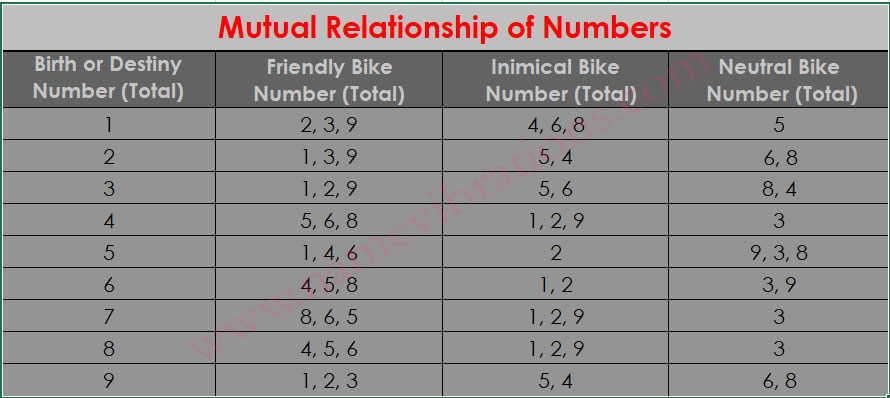+1 (705) 906-9693 contact@namevibrations.com
Select Page

## Lucky Number for Bike Calculator

What is Lucky Number for Bike?

Your Bike number should be friendly (in harmony) to either your Lucky Number or Birth Number or Destiny Number.

Enter your date of birth and prospective bike number in our Lucky Number for Bike Calculator astrological tool to match your bike number with your Birth Number or Destiny Number. We have taken both Chaldean & Pythagorean values of the bike number to get better result.

## How does the Tool work?

1. Calculates your birth number and destiny number.
2. Gets the numerical value of the alphabets.
3. Adds the numerical value of the alphabets and digits in the bike number.
4. Finally, bring that total to a single digit.

NOTE: That single-digit number should be friendly to your birth (psychic) number or destiny (life path) number.

To know the compatibility kindly refer to the Mutual Relationship of Numbers (Friendship or Enmity Chart) below and if you want to do the manual calculation please scroll down to the see the method and chalden & pythagorean numerical value of the alphabets.

## How to read the results?

There can be four instances in matching the bike number to birth number or destiny number:

1. Both the Chaldean & Pythagorean values of bike numbers are friendly to the birth number and/or destiny number. (amazing Compatibility)
2. Both the Chaldean and Pythagorean value of the bike numbers are not friendly to the birth number and/or destiny number. (worst compatibility)
3. Only the Chaldean value of the bike number is friendly or neutral but Pythagorean value of car number is neither friendly nor neutral to the birth number and/or destiny number. (good compatibility)
4. Chaldean does not match but only Pythagorean matches the birth number and/or destiny number. (low compatibility)

If you already own a bike with a number that is neutral to your DOB (birth number & destiny number) then it is still acceptable. However, if the bike number is not friendly to your DOB, then you must change the bike.### Numerical Value of Alphabets (Chaldean & Pythagorean)

Chaldean :  AIJQY = 1, BKR = 2, CGLS = 3, DMT = 4, EHNX = 5, UVW = 6, OZ = 7, FP = 8

PythagoreanAIS = 1, BKT = 2, CGU = 3, DMV = 4, EHW = 5, FOX = 6, GPY = 7, HQZ = 8, IR = 9

### How to calculate Manually (Chaldean)

Birth Number 1

Here is a vehicle number DL 03 XB 1234. So according to the above steps.

1. DL = 43 and XB = 52
2. (0+3) + (1+2+3+4)
3. (4+3) + (5+2) + (0+3) + (1+2+3+4)
4. 7 + 7 + 3 + 10 = 28 = 10 = 9

Basic rule is take the total of the numbers (1+2+3+4)  = 10 = 1

My personal experience is most of the time if total of the number is friendly (1+2+3+4)  = 10 = 1

Chances are more that entire number comes out friendly (DL XB 03 1234) = (4+3) + (5+2) + (0+3) + (1+2+3+4) = 9

Here Total of numbers = 1 & Total of entire number = 9 (Both Friendly to Birth Number 1)

In case it is not, even if you have to pay some fees or wait for some time, get a number that is friendly (in harmony) to your Birth Number.